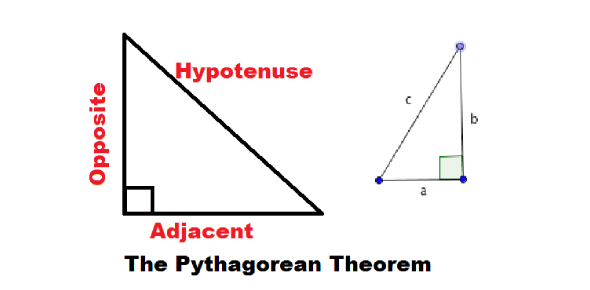How would you apply the Pythagorean Theorem to a set of complex - ProProfs DiscussTopicsMoreProductsMore+ Ask Question

# How would you apply the Pythagorean Theorem to a set of complex numbers?

Asked by K. Tanaka, Last updated: Dec 18, 2019

###Request 0FollowShareAnswer AnonymouslyAnswer LaterCopy Link#### John F. connor

Traveler, Avid Reader, Free as a bird

John F. connor, Content Marketing executive, MA, Minsk,PolandIf I had a math teacher who gave me a difficult problem because it had a set of complex numbers to use, I would definitely use a calculator. A complex number is one that includes two real numbers that are seen in the form of A and B. Then there is one that is the result of an equation. The equation is x squared is equal to negative one.This number is considered to be an imaginary number. Therefore, these complex numbers may make it difficult to calculate the Pythagorean theorem’s formula. The best way to do this is to use a special type of calculator. Some of the calculators have the higher-level things and objects on them in order to calculate the hypotenuse of a triangle.Search for Google imagesSelect a recommended image
Upload from your computerCancelSearch for Google imagesSelect a recommended image
Upload from your computerCancelSearch for Google imagesSelect a recommended image
Upload from your computerCancel